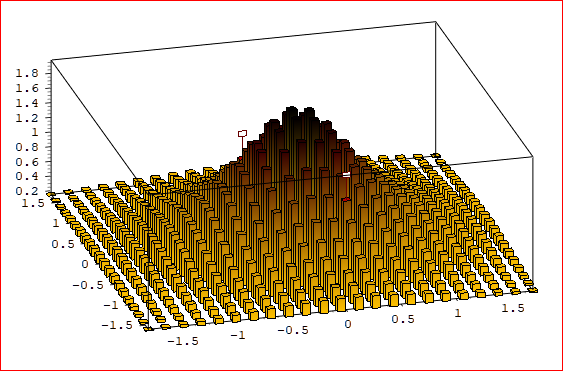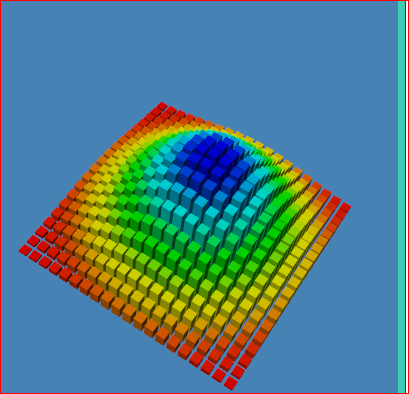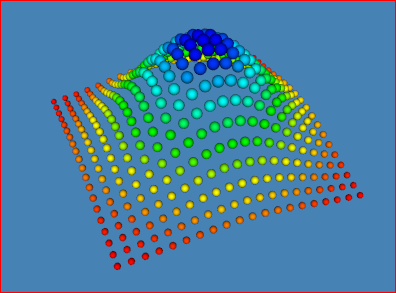# 3D bar charts in VTK?

I would like to implement a 3D bar chart similar to this (from qwt3D) or what is available in matplotlib. Is there any such class in VTK? If not can I ask for some pointers about how to get started?

Thanks.You could make the bars as individual geometries and plot with pyvista like so:

``````import numpy as np
import pyvista as pv

# Make up some data - gaussian
n = 20
x = np.linspace(-200, 200, num=n)
y = np.linspace(-200, 200, num=n)
xx, yy = np.meshgrid(x, y)
A, b = 50, 100
zz = A * np.exp(-0.5 * ((xx / b) ** 2.0 + (yy / b) ** 2.0))

s = 3
u, v = np.meshgrid(np.arange(n)*s, np.arange(n)*s)

# Make each bar
cubes = pv.MultiBlock()
for point in np.c_[u.reshape(-1), v.reshape(-1), zz.reshape(-1)]:
c = point
c[-1] /=2
bar = pv.Cube(center=c, z_length=point[-1])
cubes.append(bar)

# Plot all bars in 3D
p = pv.Plotter(notebook=0)
p.show_grid()
p.show()
``````

There are quite a number of ways to do this actually, such as with glyphing.

Take a look at pyvista to see more examples like this: docs.pyvista.org

Thanks. This is in essence what happens in qwt3D - the bars are “enhancements” drawn at the vertices of a 2D mesh. I’m looking for a C++ / VTK solution for use in a Qt-based C++ application, but maybe I can use the PyVista code for some ideas.

VTK support for 3D chart is a bit lacking, but here is the class:

https://vtk.org/doc/nightly/html/classvtkPlotSurface.html

Thanks, Mathieu. I actually have this partly working, using the approach from pyVista - create a vtkMultiBlockDataSet and add bars created from a vtkCubeSource to it. The code is a bit too messy to post, but here’s a screenshot. The nice this is that this model also works for creating 3D scatterplots - simply replace the cubes with spheres.1 Like